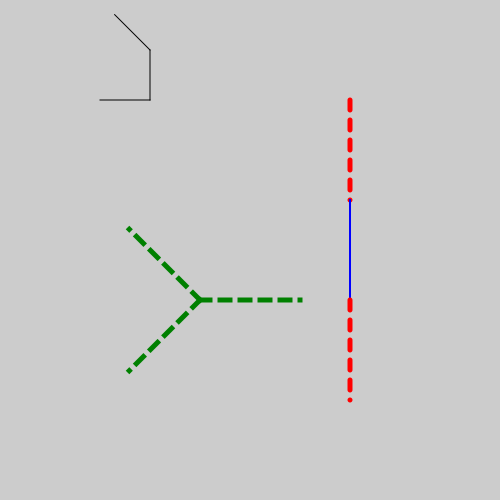# Turtles in generativepy

Martin McBride, 2022-03-07
Tags generativepy tutorial path
Categories generativepy generativepy tutorialThis tutorial covers the basics of using Turtle objects in generativepy.

This provides a simple implementation of turtle graphics. This uses an imaginary turtle that can move around, drawing a line as it goes. The basic operation of a turtle are:

• forward - move forward by a specified amount, drawing a line.
• move - move forward by a specified amount, without drawing a line.
• left, right - turn through a specified angle to the left or right.

This style of drawing allows complex geometric shapes to be drawn without needing to worry about coordinate geometry and all the trigonometry it requires.

The turtle has a few enhanced features:

• set_heading - set an absolute direction.
• move_to - move to an absolute position.
• push and pop - allows the turtle to do some drawing then return to its original position and direction. This can be done recursively, for example to draw trees.
• set_style - set the line style.

## Turtle example code

Here is a sample Python program for drawing with the turtle. The code is explained later in this article:

from generativepy.drawing import make_image, setup, ROUND
from generativepy.color import Color
from generativepy.geometry import Turtle
import math

def draw(ctx, width, height, frame_no, frame_count):
setup(ctx, width, height, background=Color(0.8))

turtle = Turtle(ctx)
turtle.move_to(100, 100).forward(50).left(math.pi / 2).forward(50).left(math.pi / 4).forward(50)

turtle = Turtle(ctx)
turtle.move_to(200, 300).set_style(Color('green'), line_width=5, dash=) \
.push().forward(100).pop() \
.push().left(3 * math.pi / 4).forward(100).pop() \
.right(3 * math.pi / 4).forward(100)

turtle = Turtle(ctx)
turtle.move_to(350, 100).right(math.pi / 2).set_style(Color('red'), line_width=5, dash=, cap=ROUND).forward(100)
turtle.set_style(Color('blue'), line_width=2, dash=[]).forward(100)
turtle.set_style(Color('black'), line_width=4, dash=).forward(100)

make_image("turtle.png", draw, 500, 500)


This code is available on github in tutorial/shapes/turtle.py.

Here is the resulting image:## Explanation

The first part of the code creates the thin, black line at the top of the image:

    turtle = Turtle(ctx)
turtle.move_to(100, 100).forward(50).left(math.pi / 2).forward(50).left(math.pi / 4).forward(50)


Here is what the code does:

• Moves to the point (100, 100),
• Draws line of length 50. The turtle initially points to the right, so the line is horizontal.
• Turns the turtle through pi/2 radians (90 degrees) left, so the turtle is pointing up the page. It then draws a line of length 50.
• Turns the turtle through pi/4 radians (45 degrees) left, so the turtle is pointing diagonally to the upper-left. It then draws a line of length 50.

Unlike other shapes, the turtle draws as it goes along, so there is no need to call stroke.

The next part of the code creates the dashed green Y shape:

    turtle = Turtle(ctx)
turtle.move_to(200, 300).set_style(Color('green'), line_width=5, dash=) \
.push().forward(100).pop() \
.push().left(3 * math.pi / 4).forward(100).pop() \
.right(3 * math.pi / 4).forward(100)


This code uses push and pop to draw three lines that all start at (200, 200):

• Moves to the start position and sets a green, dashed line style.
• Pushes. Draws a line of length 100 (in the default direction, to the right). The pops, restoring the turtle to its previous state.
• Pushes. Turns 135 degrees left, draws a line of length 100 from position (200, 200) towards the upper-left. The pops, restoring the turtle to its previous state.
• This is the last item so we don't bother pushing. Turns 135 degrees right, draws a line of length 100 from position (200, 200) towards the lower-left.

The final code illustrates styling:

    turtle = Turtle(ctx)
turtle.move_to(350, 100).right(math.pi / 2).set_style(Color('red'), line_width=5, dash=, cap=ROUND).forward(100)
turtle.set_style(Color('blue'), line_width=2, dash=[]).forward(100)
turtle.set_style(Color('black'), line_width=4, dash=).forward(100)


In this case, we draw 3 line segments, changing the line style each time - red dashed, blue solid, black dashed.

If you found this article useful, you might be interested in the book Computer Graphics in Python or other books by the same author.

#### Popular tags

2d arrays abstract data type alignment and angle animation arc array arrays bar chart bar style behavioural pattern bezier curve built-in function callable object chain circle classes clipping close closure cmyk colour combinations comparison operator comprehension context context manager conversion count creational pattern data science data types decorator design pattern device space dictionary drawing duck typing efficiency ellipse else encryption enumerate fill filter font font style for loop function function composition function plot functools game development generativepy tutorial generator geometry gif global variable gradient greyscale higher order function hsl html image image processing imagesurface immutable object in operator index inner function input installing iter iterable iterator itertools join l system lambda function len lerp line line plot line style linear gradient linspace list list comprehension logical operator lru_cache magic method mandelbrot mandelbrot set map marker style matplotlib monad mutability named parameter numeric python numpy object open operator optimisation optional parameter or pandas partial application path pattern permutations pie chart polygon positional parameter print pure function python standard library radial gradient range recipes rectangle recursion reduce regular polygon repeat rgb rotation roundrect scaling scatter plot scipy sector segment sequence setup shape singleton slice slicing sound spirograph sprite square str stream string stroke structural pattern subpath symmetric encryption template text text metrics tinkerbell fractal transform translation transparency triangle truthy value tuple turtle unpacking user space vectorisation webserver website while loop zip